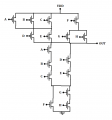# Circuit diagram of CMOS function

#### J_Rod

Joined Nov 4, 2014
109
Good morning,
In this problem given is a logical function Y = [ (ABC +DE)(F +GH) ]' and I am supposed to draw the CMOS circuit implementing this. I know the pull-down path is correct but I am not sure if the pull-up path is correct.For the pull-down path, OUT = 0 when A=B=C=F = 1, A=B=C=G=H = 1, D=E=F = 1, and D=E=G=H = 1. This means the transistors will be in the linear mode of operation and will pull down OUT to ground. For the pull-up path, the general rule is that transistors in series in the pull-down path become in parallel, and transistors in parallel in the pull-down network become in series. If A or B or C and E = 0, OUT = 1. If A or B or C and D = 0, OUT = 1. If F and G = 0, OUT = 1. If F and H = 0, OUT = 1. Thanks for the help.

#### WBahn

Joined Mar 31, 2012
26,910
The pullup and pulldown paths are complements of each other, so DeMorgans theorem dictates that whatever is in parallel in one will be in series in the other and vice-versa.

Your circuit looks fine (assuming I'm not missing something -- always a possibility).

•J_Rod